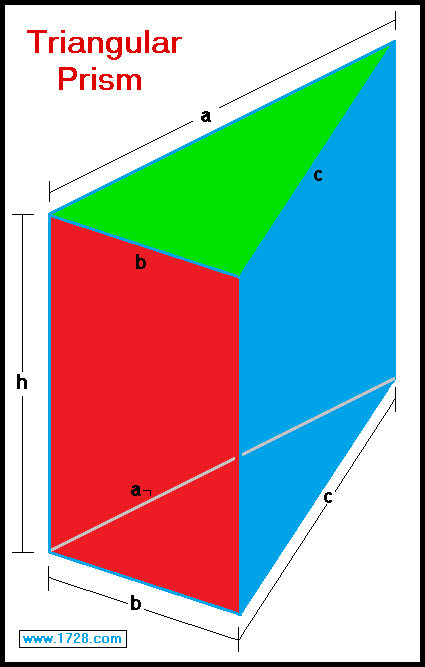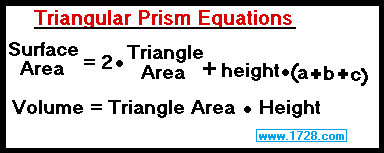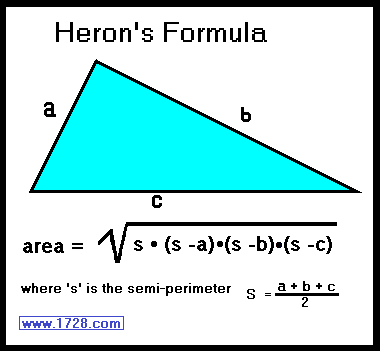Triangular Prism CalculatorInput the 3 triangle sides and the height:

>>>>>
>>>>>
>>>>>
>>>>>

If you need the area in greater detail, please use this information:

 A triangular prism is a geometric solid having 2 identical, parallel, triangular bases which are perpindicular to the 3 rectangular faces. In the above graphic, the green triangle is one of the bases and the red and blue planes are 2 of the 3 faces. The triangles can be any type of triangle (acute, right or obtuse). This calculator computes a triangular prism's area and volume when the triangle sides and prism height have been input. It's great to have a calculator for calculating triangular prisms, but let's see the mathematics of this.

 A triangular prism has sides of 5, 6 and 7 with a height of 9. What is the area and volume of this prism? The first thing to do is to calculate the area of the triangular base. Since we know all 3 triangle sides, we will us Heron's Formula. Semi-Perimeter = (5 + 6 + 7) ÷ 2 Semi-Perimeter = 18 ÷ 2 Semi-Perimeter = 9 Triangle Area = square root [9 * (9-5) * (9-6) * (9-7)] Triangle Area = square root [9 * 4 * 3 * 2] Triangle Area = square root (216) Triangle Area = 14.6969384567 Since a triangular prism has two triangles, the area of both triangles equals 2 * 14.6969384567 = 29.3938769134
 Now to calculate the area of the 3 rectangular faces: Rectangular face area = 9 * (5 + 6 + 7) Rectangular face area = 162 For the total prism area we add up both areas: Total Area = 29.3938769134 + 162 Total Area = 191.3938769134 Since we already calculated the triangle area, the volume is easily calculated by: Volume = triangle area * height Volume = 14.6969384567 * 9 Volume = 132.2724461103

Significant Figures >>>
Numbers are displayed in scientific notation with the amount of significant figures you specify. For easier readability, numbers between .001 and 1,000 will not be in scientific notation but will still have the same precision.
You may change the number of significant figures displayed by changing the number in the box above.
Most browsers, will display the answers properly but if you are seeing no answers at all, enter a zero in the box above, which will eliminate all formatting but at least you will see the answers.